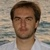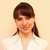# Posts tagged "Calculations"## How to calculate standard error of the mean in Excel

Whether you are a student, researcher, or data analyst, understanding the standard error of the mean can help you draw more accurate conclusions from your data and make more informed decisions. This article will show you a few quick ways to calculate the standard error in Excel. Continue reading## Calculating percent change (percentage increase / decrease) in Excel

In Microsoft Excel, there are 6 different functions for calculating variance but all of them are designed to find variance in the classical sense, i.e. how far a set of values are spread out from their average. In this article, you will learn how to calculate percentage variance between two cells. Continue reading## How to subtract in Excel: formula for numbers, percentages, dates and times

Subtraction is one of the four basic arithmetic operations, and every primary school pupil knows that to subtract one number from another you use the minus sign. This good old method also works for subtracting numbers and cells in Excel. Continue reading## How to divide in Excel and handle #DIV/0! error

As with other basic math operations, Microsoft Excel provides several ways to divide numbers and cells. Which one to use depends on your personal preferences and a particular task you need to solve. Continue reading## How to multiply columns in Excel

As with all basic arithmetic operations, there is more than one way to multiply columns in Excel. This tutorial will show you a few possible solutions so you can choose the one that works best for you. Continue reading## How to multiply cells in Excel with formula examples

This tutorial shows how to use the multiplication operator in Excel and how to write a formula to multiply cells, columns and rows, numbers and percentages, and more. Continue reading## How to do calculations in Excel

When it comes to calculations, there is almost noting that Microsoft Excel cannot do, from totaling a column of numbers to solving complex linear programming problems. Apart from that, you can use Excel as a calculator to do math - add, divide, multiply, and subtract numbers as well as raise to power and find roots. Continue reading## How to square root in Excel: SQRT function and other ways

Squaring a number and finding a square root are very common operations if mathematics. But how do you do square root in Excel? Either by using the SQRT function or by raising a number to the power of 1/2. Continue reading## Excel Cumulative Sum - easy way to calculate running total

This short tutorial shows how a usual Excel Sum formula with a clever use of absolute and relative cell references can quickly calculate a running total in your worksheet. Continue reading## How to calculate weighted average in Excel

The tutorial demonstrates two easy ways to calculate weighted average in Excel - by using the SUM or SUMPRODUCT function. Continue reading